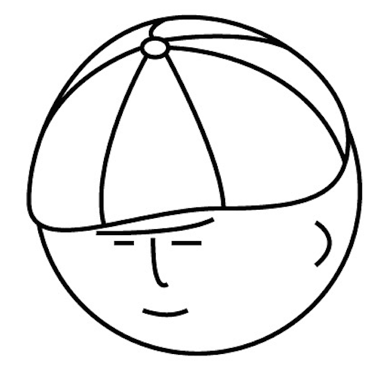# マツシタのお勉強メモ

#### マツシタのお勉強

##### welcome to my engineering blog
###### Hacker Rank# ■

Problem leetcode.com Solution This problem can be solve by DFS with depth of tree. In my code, lists.size() == depth means that the traversing depth changes. Source Code

# Heap Sort in Java

What is Heap Reference www.youtube.com Heap is the tree structure that has two following restrictions. Binary Tree Parent node is smaller or bigger than children nodes. Heap is good at controlling the relation about small and large. So hea…

# Print all paths which sum to a given value in a Binary Tree

Problem You are given a binary tree in which each node contains a value. Design an algorithm to print all paths which sum to a given value. The path dose not need to start or end at the root or leaf. Solution First, let’s approach this pro…

# Check if small binary tree T1 is a suibtree of large binary Tree T2

Problem You have two large binary tree: T1, with millions of nodes, and T2, with hundreds of nodes. Create an algorithm to decide if T2 is a subtree of T1. A tree T2 is a subtree of T1 if there exists a node n in T1 such the the subtree of…

# Find the First Common Ancestor of the two nodes in a binary tree

Problem Design an algorithm and write code to find the first common ancestor of two nodes in a binary tree. Avoid storing additional nodes in a data structure. Node: This is not necessarily a binary search tree. Solution I’ll solve this pr…

# Create a Linked List of all the nodes at each depth in Binary Tree

Problem Given a binary tree, design a algorithm which creates a linked list of all the nodes at each depth (e.g, if you have a tree with depth D, you'll have D linked lists) How to Solve The important point of the this problem How we shoul…

# Check if a binary tree is balanced

Problem Implement a function to check if a binary tree is balanced. For the purposes of this question. balanced tree is defined to be a tree such that the heights of the two subtrees of any node never differ by more than one. How to Solve …

# 298. Binary Tree Longest Consecutive Sequence : Past Google Coding Interview

Problem https://leetcode.com/problems/binary-tree-longest-consecutive-sequence/ How to Solve This problem is classified into Tree and can be solve by using DFS(Depth First Search). Depth First Search Firstly, I thought the only search by D…